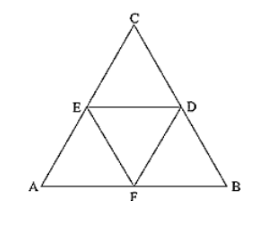# In a ΔABC, ∠A = 50°, ∠B = 60° and ∠C = 70°.

Question:

In a ΔABC, ∠A = 50°, ∠B = 60° and ∠C = 70°. Find the measures of the angles of the triangle formed by joining the mid-points of the sides of this triangle.

Solution:

In ΔABC,

D and E are mid points of AB and BC.

By Mid point theorem,

DE ∥ AC, DE = (1/2) AC

F is the midpoint of AC

Then, DE = (1/2) AC = CF

DE ∥ AC, DE = CF

Hence DECF is a parallelogram.

∴ ∠C = ∠D = 70°        [Opposite sides of a parallelogram]

Similarly

BEFD is a parallelogram, ∠B = ∠F = 60°

ADEF is a parallelogram, ∠A = ∠E = 50°

∴ Angles of ΔDEF are

∠D = 70°, ∠E = 50°, ∠F = 60°